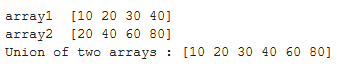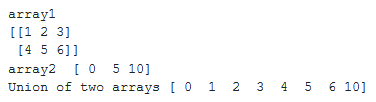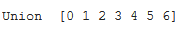# Find the union of two NumPy arrays

• Last Updated : 05 Sep, 2020

To find union of two 1-dimensional arrays we can use function numpy.union1d() of Python Numpy library. It returns unique, sorted array with values that are in either of the two input arrays.

Syntax:

Attention geek! Strengthen your foundations with the Python Programming Foundation Course and learn the basics.

To begin with, your interview preparations Enhance your Data Structures concepts with the Python DS Course. And to begin with your Machine Learning Journey, join the Machine Learning - Basic Level Course

```numpy.union1d(array1, array2)
```

Note The arrays given in input are flattened if they are not 1-dimensional.

Let’s see examples of how to find union of two arrays.

Example 1:

 `# import libraries``import` `numpy as np`` ` ` ` `arr1 ``=` `np.array([``10``, ``20``, ``30``, ``40``])``print``(``"array1 "``, arr1)`` ` `arr2 ``=` `np.array([``20``, ``40``, ``60``, ``80``])``print``(``"array2 "``, arr2)`` ` `# print union of the two arrays``print``(``"Union of two arrays :"``, np.union1d(arr1, arr2))`

Output:Example 2:
Let’s see example of finding union of a 2-d and a 1-d array. As discussed earlier, if array passed as arguments to function numpy.union1d is 2-dimensional, then they are flattened to 1-dimension.

 `# import libraries``import` `numpy as np`` ` ` ` `# 2-d array``arr1 ``=` `np.array([[``1``, ``2``, ``3``], [``4``, ``5``, ``6``]])``print``(``"array1 "``)``print``(arr1)`` ` `arr2 ``=` `np.array([``0``, ``5``, ``10``])``print``(``"array2 "``, arr2)`` ` `# print union of 2-d array and 1-d array``print``(``"Union of two arrays"``, np.union1d(arr1, arr2))`

Output:Example3:
If we want to find union of more than two arrays, then we can find that by using functools.reduce function.

 `# code to find union of more than two arrays``# import libraries``import` `numpy as np``from` `functools ``import` `reduce`` ` ` ` `array ``=` `reduce``(np.union1d, ([``1``, ``2``, ``3``], [``1``, ``3``, ``5``],``                            ``[``2``, ``4``, ``6``], [``0``, ``0``, ``0``]))``print``(``"Union "``, array)`

Output:My Personal Notes arrow_drop_up